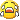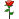Question:

Given x^2 - y^2 = 1001

(a) Prove that x is divisible by 3.
(b) Solve x and y.

x= 75 and y= 68

Sorry sorry

Try reasoning with modulus.

You may also prove that x is odd and y is even

Solution for Part (a):

1001MOD3 = 2
Since for all real square n,
##### 引用:
nMOD3 =/= 2

So (y^2)MOD3 = 1 and (x^2)MOD3 = 0

Hence, x^2 must be odd
Since 1001 is odd, y^2 must be even.

Solution to Part (b):

(x+y)(x-y) = 1001

Since 1001 = 7 x 11 x 13

You can exhaust all x and y then.

##### 引用:

Solution to Part (b):

(x+y)(x-y) = 1001

Since 1001 = 7 x 11 x 13

You can exhaust all x and y then.

I don't know how use modIt was out of syllabus at my time.

All I know is to exhaust it. I called it brute force method.

Using mod in (a) is obviously more elegant!

Thank you so much for teaching me!

Maths is so fun